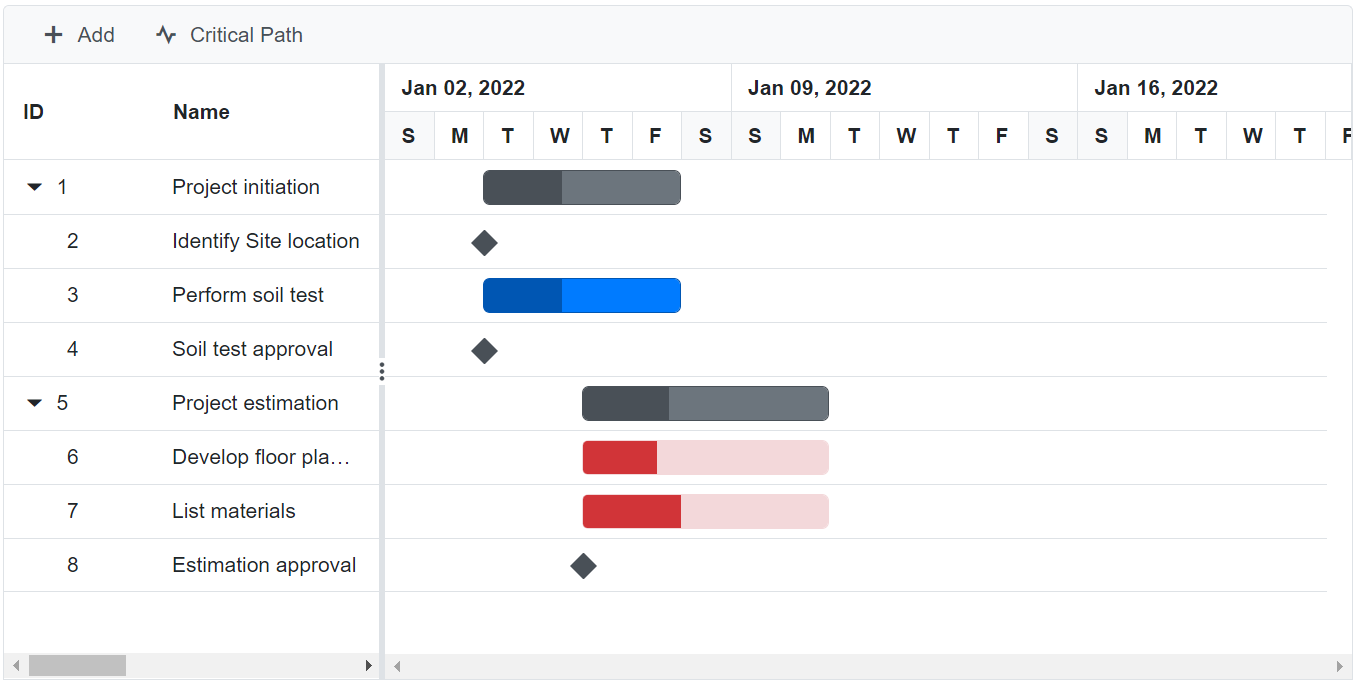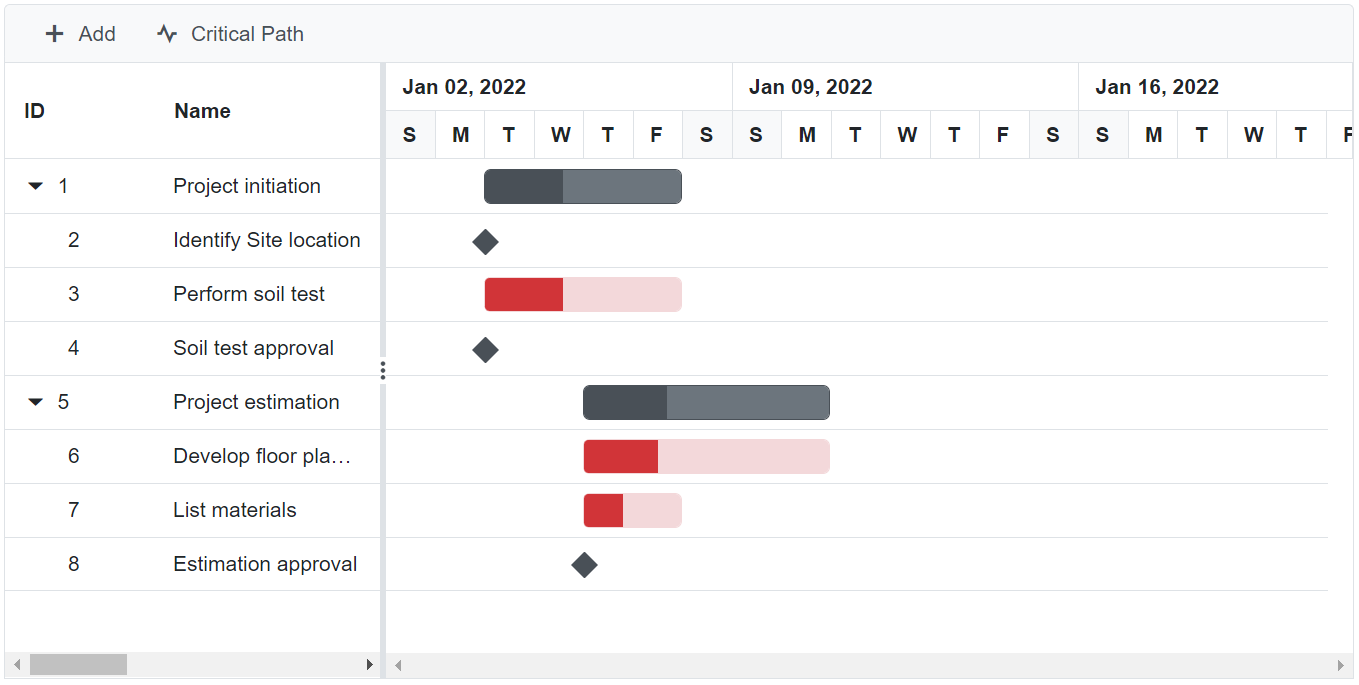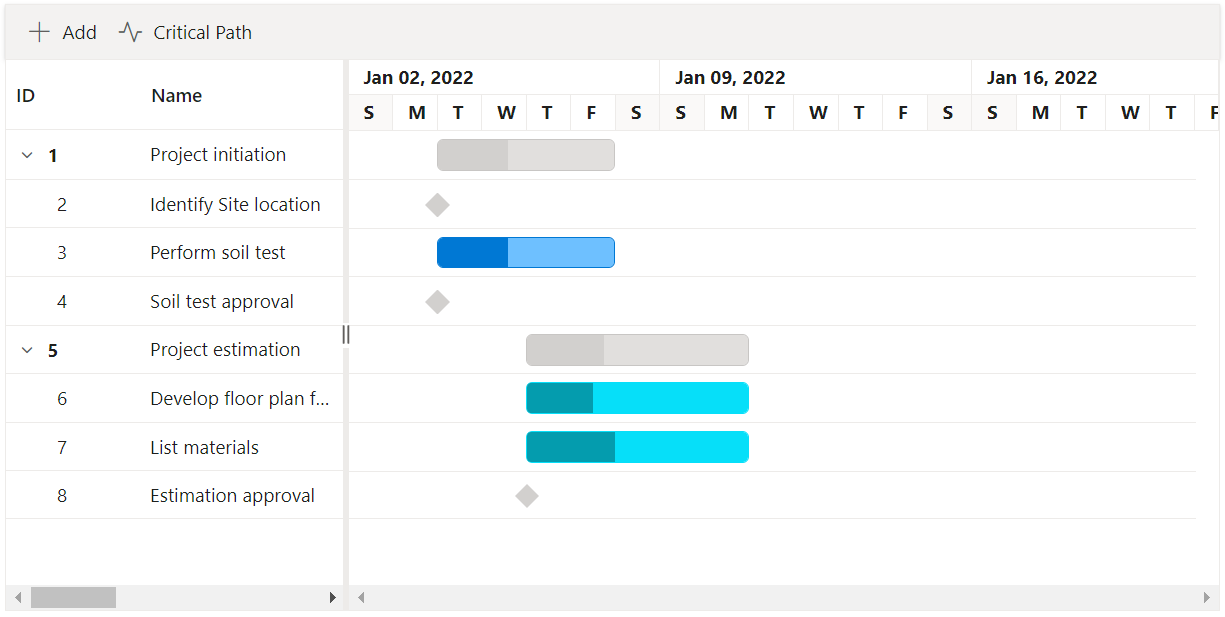# Critical Path in Blazor Gantt Chart component

22 Nov 202311 minutes to read

The critical path in a project is indicated by a single task or a series of tasks. If a task in critical path is delayed, the entire project will be delayed. A task is considered to be critical if any delay to this task would affect the project end date.

The critical path can be enabled in Gantt by using the built-in toolbar button or EnableCriticalPath property.

The following code example shows how to display the critical path in the Gantt control:

``````@using Syncfusion.Blazor.Gantt
<SfGantt DataSource="@TaskCollection" EnableCriticalPath Toolbar="@(new List<string>() { "Add", "Cancel", "Delete", "Edit","CriticalPath" })" Height="450px" Width="900px">
Duration="Duration" Progress="Progress" ParentID="ParentId">
</GanttEditSettings>
</SfGantt>

@code{
protected override void OnInitialized()
{
}

{
public int TaskId { get; set; }
public string TaskName { get; set; }
public DateTime StartDate { get; set; }
public DateTime EndDate { get; set; }
public string Duration { get; set; }
public int Progress { get; set; }
public int? ParentId { get; set; }
}

{
{
new TaskData() { TaskId = 1, TaskName = "Project initiation", StartDate = new DateTime(2022, 01, 04), EndDate = new DateTime(2022, 01, 17), },
new TaskData() { TaskId = 2, TaskName = "Identify Site location", StartDate = new DateTime(2022, 01, 04), Duration = "0", Progress = 30, ParentId = 1, },
new TaskData() { TaskId = 3, TaskName = "Perform soil test", StartDate = new DateTime(2022, 01, 04), Duration = "4", Progress = 40, ParentId = 1, },
new TaskData() { TaskId = 4, TaskName = "Soil test approval", StartDate = new DateTime(2022, 01, 04), Duration = "0", Progress = 30, ParentId = 1, },
new TaskData() { TaskId = 5, TaskName = "Project estimation", StartDate = new DateTime(2022, 01, 04), EndDate = new DateTime(2022, 01, 17), },
new TaskData() { TaskId = 6, TaskName = "Develop floor plan for estimation", StartDate = new DateTime(2022, 01, 06), Duration = "3", Progress = 30, ParentId = 5, },
new TaskData() { TaskId = 7, TaskName = "List materials", StartDate = new DateTime(2022, 01, 06), Duration = "3", Progress = 40, ParentId = 5, },
new TaskData() { TaskId = 8, TaskName = "Estimation approval", StartDate = new DateTime(2022, 01, 06), Duration = "0", Progress = 30, ParentId = 5, }
};
}
}``````## Critical path settings in Gantt Chart Component

The critical path settings in the Gantt Chart component determine the default slack value and validate it. Here is an example of how to add slack value to the Gantt Chart component.

Slack is a measure of how many days a task can be delayed without affecting the project’s completion. By default, tasks with zero or negative slack values are critical, while tasks with positive slack values are non-critical. If a task’s end date is the same as the project’s end date, the slack value is 0, and the task is considered critical.

You can change the default behavior of critical tasks by using the slackValue property of `GanttCriticalPathSettings`. This property defines how many days a task can be delayed before it is considered critical. For instance, in the following code example, tasks with two days of slack are shown on the critical path, so potential issues can be detected earlier.

By adjusting the slackValue, you can control which tasks are critical and ensure that potential issues are identified and addressed in a timely manner.

``````@using Syncfusion.Blazor.Gantt
<SfGantt DataSource="@TaskCollection" EnableCriticalPath Toolbar="@(new List<string>() { "Add", "Cancel", "Delete", "Edit","CriticalPath" })" Height="450px" Width="900px">
Duration="Duration" Progress="Progress" ParentID="ParentId">
</GanttEditSettings>
<GanttCriticalPathSettings SlackValue="2" ></GanttCriticalPathSettings>
</SfGantt>

@code{
protected override void OnInitialized()
{
}

{
public int TaskId { get; set; }
public string TaskName { get; set; }
public DateTime StartDate { get; set; }
public DateTime EndDate { get; set; }
public string Duration { get; set; }
public int Progress { get; set; }
public int? ParentId { get; set; }
}

{
{
new TaskData() { TaskId = 1, TaskName = "Project initiation", StartDate = new DateTime(2022, 01, 04), EndDate = new DateTime(2022, 01, 17), },
new TaskData() { TaskId = 2, TaskName = "Identify Site location", StartDate = new DateTime(2022, 01, 04), Duration = "0", Progress = 30, ParentId = 1, },
new TaskData() { TaskId = 3, TaskName = "Perform soil test", StartDate = new DateTime(2022, 01, 04), Duration = "4", Progress = 40, ParentId = 1, },
new TaskData() { TaskId = 4, TaskName = "Soil test approval", StartDate = new DateTime(2022, 01, 04), Duration = "0", Progress = 30, ParentId = 1, },
new TaskData() { TaskId = 5, TaskName = "Project estimation", StartDate = new DateTime(2022, 01, 04), EndDate = new DateTime(2022, 01, 17), },
new TaskData() { TaskId = 6, TaskName = "Develop floor plan for estimation", StartDate = new DateTime(2022, 01, 06), Duration = "3", Progress = 30, ParentId = 5, },
new TaskData() { TaskId = 7, TaskName = "List materials", StartDate = new DateTime(2022, 01, 06), Duration = "2", Progress = 40, ParentId = 5, },
new TaskData() { TaskId = 8, TaskName = "Estimation approval", StartDate = new DateTime(2022, 01, 06), Duration = "0", Progress = 30, ParentId = 5, }
};
}
}``````## Customize taskbar in critical path

The taskbar in critical path can be customized by using QueryChartRowInfo event. The GanttTaskModel in the event argument is used to retrieve taskbar information.

The following code example shows how to customize the critical path taskbar in the Gantt control:

``````@using Syncfusion.Blazor.Gantt

<SfGantt DataSource="@TaskCollection" EnableCriticalPath="true" Toolbar="@(new List<string>() { "Add", "Cancel", "Delete", "Edit","CriticalPath" })" Height="450px" Width="900px">
Duration="Duration" Progress="Progress" ParentID="ParentId">
</GanttEditSettings>
</SfGantt>

@code {
protected override void OnInitialized()
{
}

{
{
}
}

{
public int TaskId { get; set; }
public string TaskName { get; set; }
public DateTime StartDate { get; set; }
public DateTime EndDate { get; set; }
public string Duration { get; set; }
public int Progress { get; set; }
public int? ParentId { get; set; }

}

{
{
new TaskData() { TaskId = 1, TaskName = "Project initiation", StartDate = new DateTime(2022, 01, 04), EndDate = new DateTime(2022, 01, 7), },
new TaskData() { TaskId = 2, TaskName = "Identify Site location", StartDate = new DateTime(2022, 01, 04), Duration = "0", Progress = 30, ParentId = 1, },
new TaskData() { TaskId = 3, TaskName = "Perform soil test", StartDate = new DateTime(2022, 01, 04), Duration = "4", Progress = 40, ParentId = 1, },
new TaskData() { TaskId = 4, TaskName = "Soil test approval", StartDate = new DateTime(2022, 01, 04), Duration = "0", Progress = 30, ParentId = 1, },
new TaskData() { TaskId = 5, TaskName = "Project estimation", StartDate = new DateTime(2022, 01, 04), EndDate = new DateTime(2022, 01, 7), },
new TaskData() { TaskId = 6, TaskName = "Develop floor plan for estimation", StartDate = new DateTime(2022, 01, 06), Duration = "3", Progress = 30, ParentId = 5, },
new TaskData() { TaskId = 7, TaskName = "List materials", StartDate = new DateTime(2022, 01, 06), Duration = "3", Progress = 40, ParentId = 5, },
new TaskData() { TaskId = 8, TaskName = "Estimation approval", StartDate = new DateTime(2022, 01, 06), Duration = "0", Progress = 30, ParentId = 5, }
};